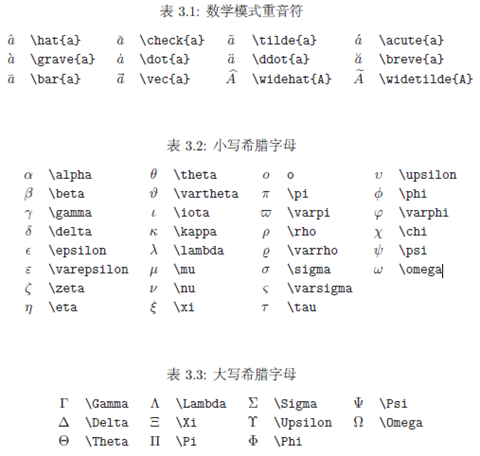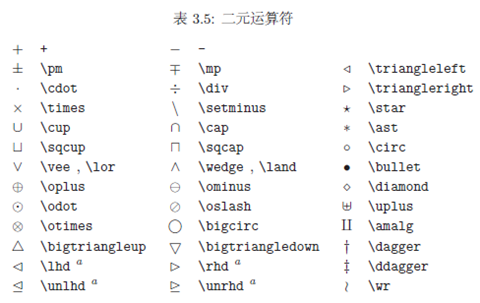## Introduction to LaTeX

LaTeX is a powerful typesetting tool that is rarely touched by the general scene, but is very much used in the field of mathematics, mainly because he can generate various mathematical formulas very easily. To learn how to use LaTeX you only need to learn a few simple concepts.Display Mode

Display mode mainly controls whether you need to display the formula content between the body lines or on a separate line, using a very simple method, that is, the company content into the $symbol among. • Intra-line mode:$ … $• Interline mode: $$…$$ Subscripts Use ^ for superscript and _ for subscript. It works by default on only one character after that, if you want to work on several consecutive characters, enclose those characters in brackets {}, which is the concept of grouping below. Grouping Grouping is the use of {} to contain the content as a whole, for example when the subscripts are long. When you encounter when the result you get is not what you expected, then most likely you need to add a grouping, i.e. add a curly bracket. Identifying letters There are often Greek letters that appear in mathematical formulas. To display these Greek letters, simply use a simple English word escape, and the common identifying letters are as follows.Arithmetic symbols The operation symbols are similar to the identification letters, any symbols that the keyboard cannot represent directly can be implemented in an escaped way. Common symbols are as follows.## From WP QuickLaTeX to MathJax-LaTeX Since your blog involves a lot of algorithmic formulas, you need to insert mathematical formulas into your articles. The easier way is to install the LaTeX plugin. The blog started out using WP QuickLaTeX, but found a major design flaw: the performance got slower and slower as the volume of posts (number of formulas used) increased. The reason for this is that WP QuickLaTeX generates images of the formulas in advance, which are stored in /wp-content/ql-cache, and I looked at my My directory, which generated a total of 15912 files. This makes finding the files very, very slow and slows down the overall speed of the site. In addition, each time an article is published (if it contains a lot of formulas) the generated images are taking up a lot of CPU resources. MathJax-LaTeX only saves LaTeX syntax. The exact rendering is performed by the front-end. So there are no problems caused by generating a large number of images. Problems encountered in migration of WP QuickLaTeX to MathJax-LaTeX. Problems encountered during migration. Enabling tags differently WP QuickLaTeX is enabled in the article using the [ latexpage ] tag. And MathJax-LaTeX with the tag [ mathjax] Solution: Change the shortcode definition in the middle of class-mathjax-latex.php  1 2  // add_shortcode( 'mathjax', [ __CLASS__, 'mathjax_shortcode' ] ); add_shortcode( ' latexpage ', [ __CLASS__, 'mathjax_shortcode' ] );  MathJax-LaTeX does not support an in-line formula i.e. the syntax representation of a$ sign is not supported

Solution: Add the following code to the MathJax code before it is loaded.

  1 2 3 4 5 6 7 8 9 10  // * 新增代码开始 // * 新增代码结束